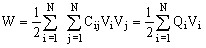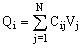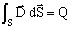# Computation of capacitance matrices

## Introduction

This paragraph explains the capacitance computation and capacitance matrix. It provides a short reminder on the equations. Then it presents two methods:

• a traditional method that computes the power balance and requires N.(N+1)/2 finite element computations

• a more original method that requires an inversion of a NxN matrix, but needs only N finite element computations

## Principle

In the presence of N conductors it is necessary to determine the capacitance of each conductor (Cii) and the “mutual” capacitance (Cij) corresponding to the interactions between various conductors. It is then necessary to find out NxN coefficients for N conductors. Fortunately, the interaction of conductor i on conductor j is equal to the interaction of conductor j on conductor i, so the number of unknown factors to compute is divided by two. The number of unknown factors to be determined is then N.(N+1)/2

Energy (W) in the finite element domain is defined by:Charge (Qi) of component i is defined by:## Gauss's theorem

The Gauss's theorem computes the charge from a surface integral (any surface including the conductor):This integral can be easily computed in 2D plane using a linear integral of the normal component of the electric flux density. The integral value of this curve multiplied by the length directly gives the electric charge in Coulomb.

## Energy method

The first method is based on energy computation. Energy is computed and enables to determine a matrix coefficient for each configuration. It is then necessary to compute N.(N+1)/2 configurations.

• Step 1: Vi = 1; Vj (j different from i) = 0

Cii = 2. W / Vi2

• Step 2: Vi = 1 ; Vj = -1 ; Vk (k different from i and j) = 0

Cij = (0.5).(Cii +Cjj)-Wij

## Floating potential method

This method requires only N computations. On the other hand, it is necessary to have the possibility to invert a NxN matrix.

The principle consists in putting floating conditions on all the conductors, to impose then a constant and identical potential (Q0) on all the conductors, one by one.

This condition satisfies rather easily by a parametric computation where each conductor, defined by a line region, is assigned to a zero charge. Within computation the all charges are parametrized with a zero value as reference value and the charge Q0 as second value. Computation is mono-parametric and so N computations are carried out.

It is necessary to get the Vi values obtained on each conductor in post-processing. A matrix is thus defined with the N values of Vi obtained on each computation. The inverse matrix, multiplied by Q0 gives the capacitance matrix.

Note: If Q0 is equal 1nC, then the matrix of capacitances is directly in nF.
Note: A conductor with floating boundary conditions has a null electric charge